# Grade 6 Math STAAR Review Activities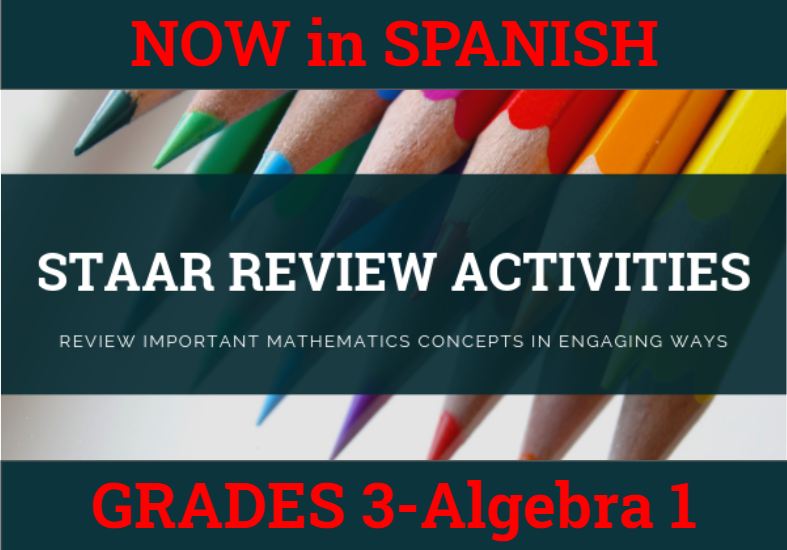How might we purposefully and effectively review important mathematics concepts for the Grade 7 Math STAAR assessment? The ESC-18 Math Team has created a variety of activities where students practice and apply important grade-level TEKS aligned topics to cement their learning.

### Please See Our Pricing and Instructions to Purchase our STAAR Activities

 6.2D Order Rational Numbers Card Sets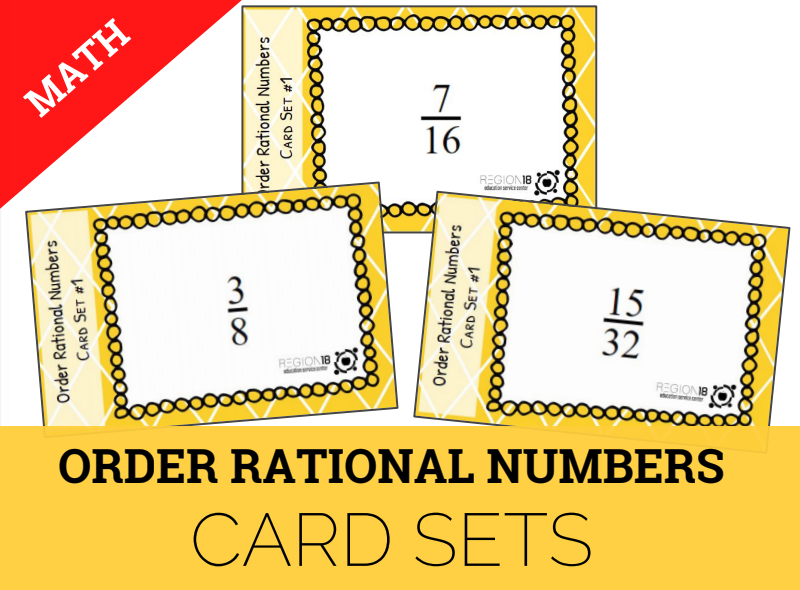Order sets of rational numbers, including fractions, decimals, and percents. 6.3D Integer Operations Stacking Pyramid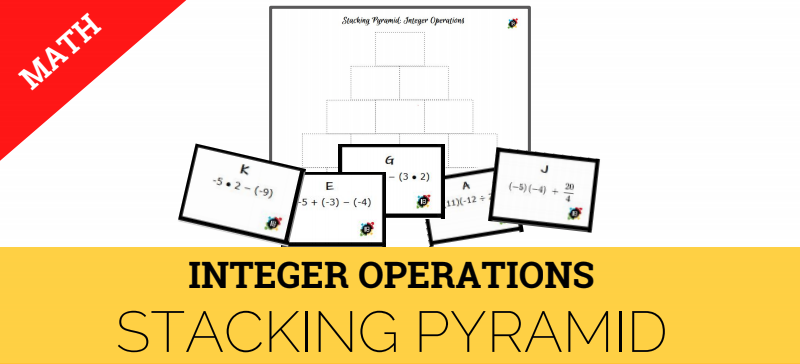Add, subtract, multiply, and divide integers fluently. 6.4B Proportional Reasoning Question Stack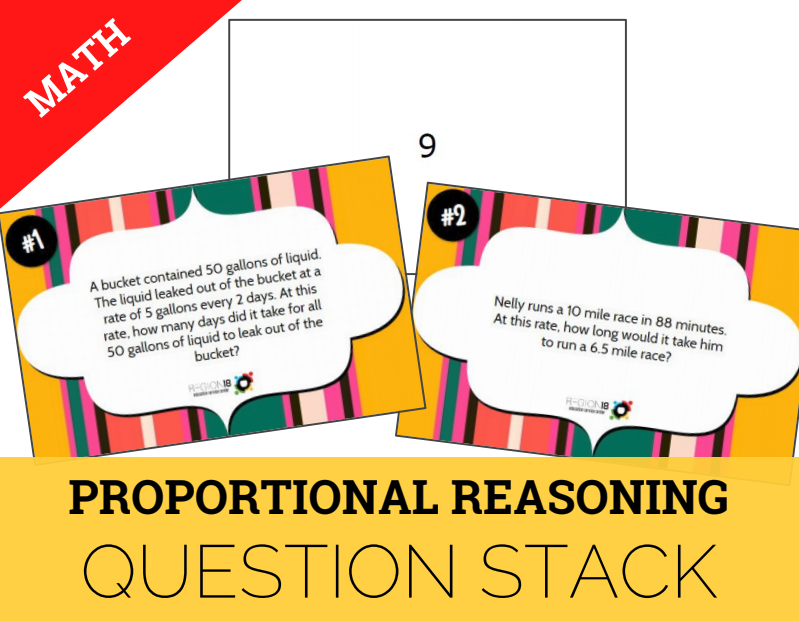Solve problems involving ratios, rates, and percents including multi-step problems involving percent increase and percent decrease, and financial literacy problems. 6.4G, 6.4E, 6.4F, 6.5C Fraction, Decimal, and Percent Card Sort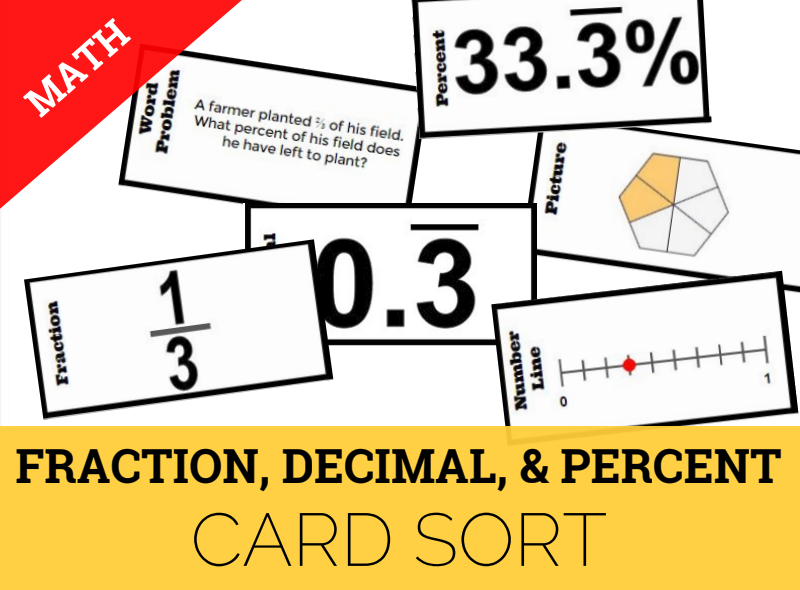Generate and represent equivalent forms of fractions, decimals, and percents, including with pictorial models and number lines. 6.5B Percent of a Number Dominoes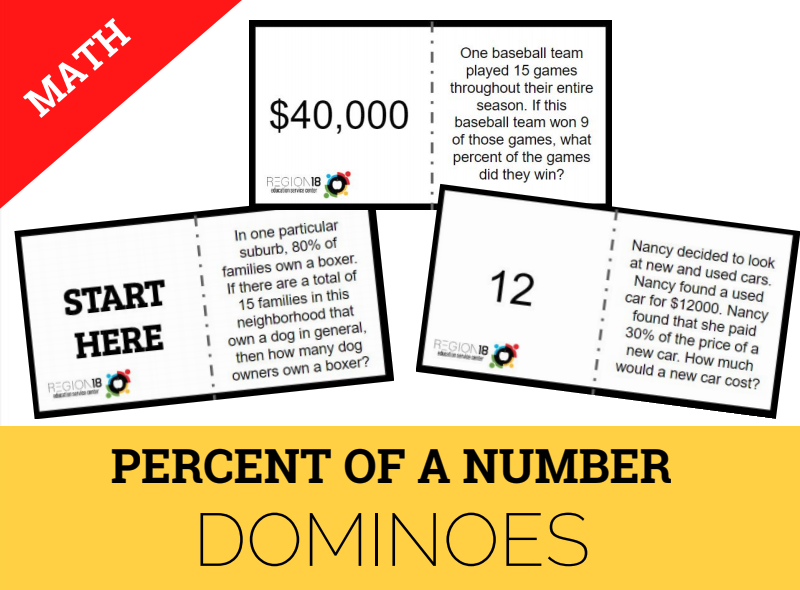Solve real-world problems to find the whole given a part and the percent, to find the part given the whole and the percent, and to find the percent given the part and the whole. 6.6C Tables, Graphs, and Equations Scavenger Hunt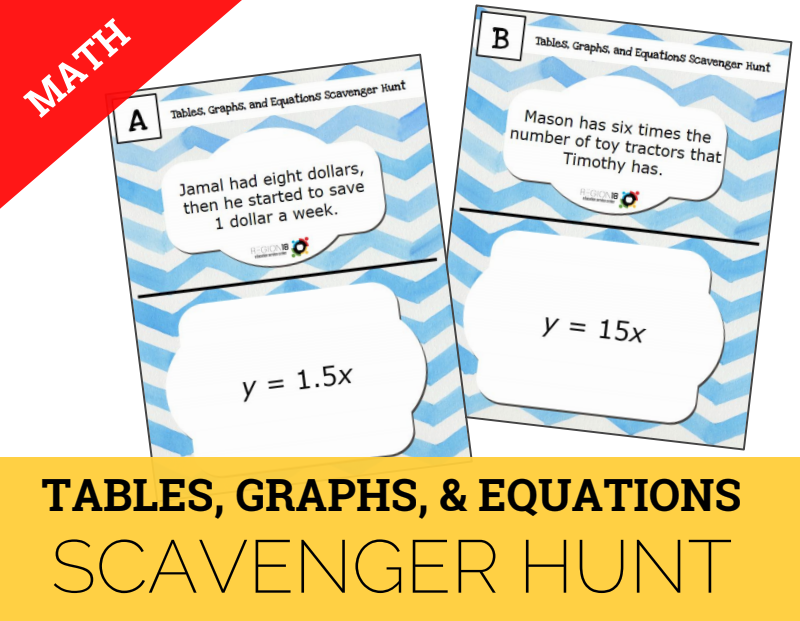Represent given situations using verbal descriptions, tables, graphs, and equations in the form y = kx or y = x + b. 6.7A, 6.7D Order of Operations Quick Flips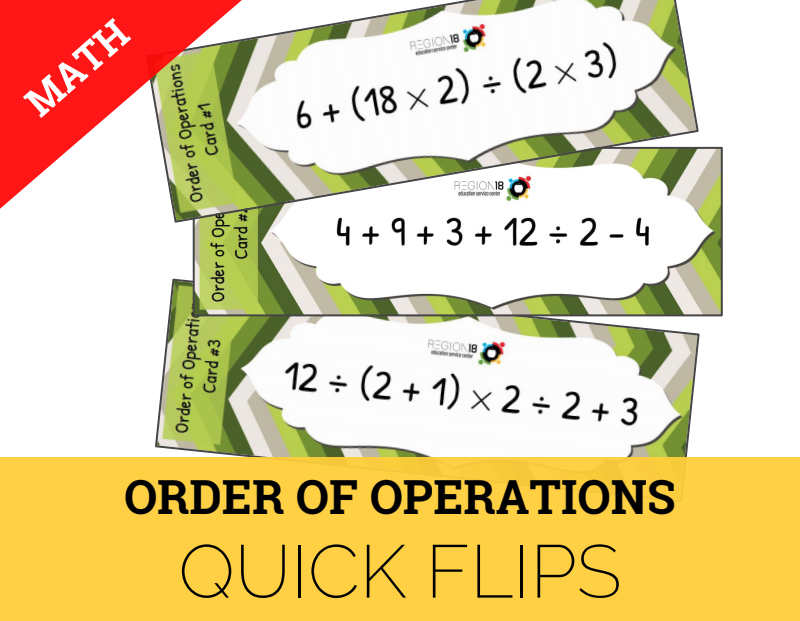Generate equivalent numerical expressions using order of operations and the properties of operations. 6.8D Area of Parallelograms, Triangles, & Trapezoids Sum It Up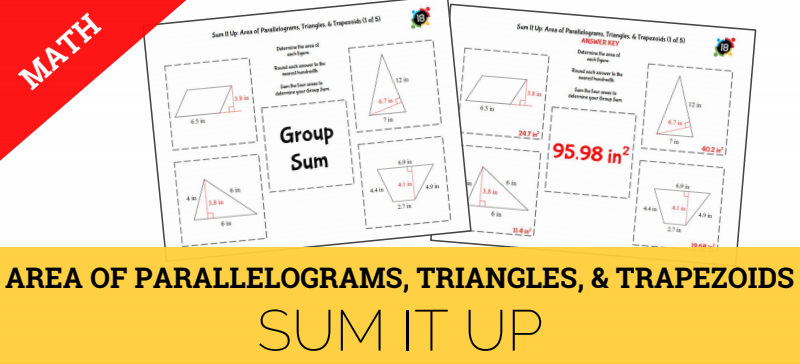Solve problems involving the area of parallelograms, trapezoids, and triangles. 6.10A Model & Solve One-Step Equations Card Sets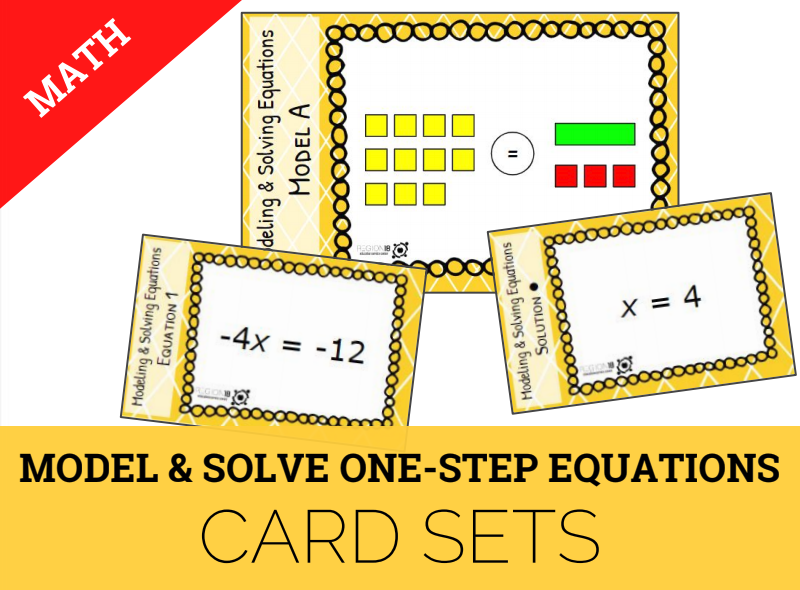Model and solve one-variable, one-step equations. 6.11A Graphing Ordered Pairs Pennants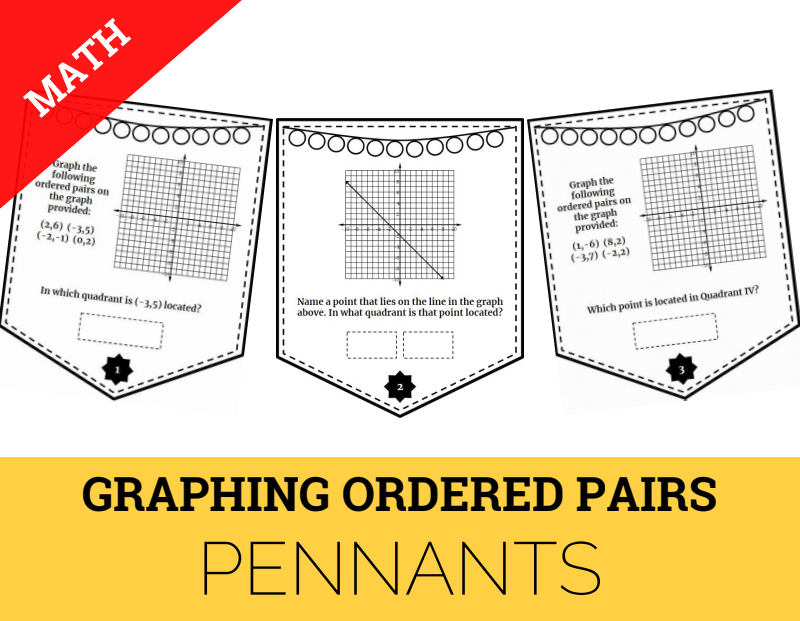Graph points in all four quadrants using ordered pairs or rational numbers. 6.13A Interpreting Numeric Data True/False Sort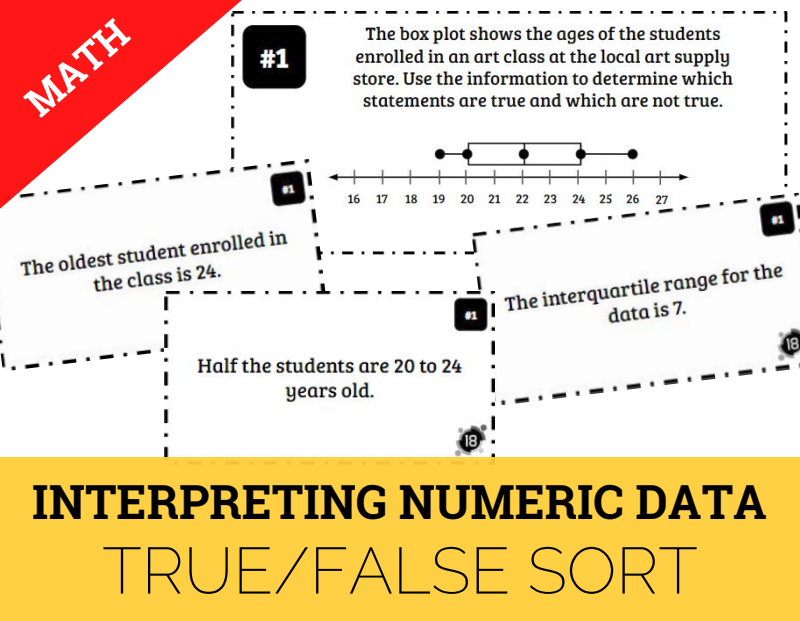Interpret numeric data summarized in dot plots, stem-and-leaf plots, histograms, and box plots. Mixed Review Grade 6 Griddable Practice Task Cards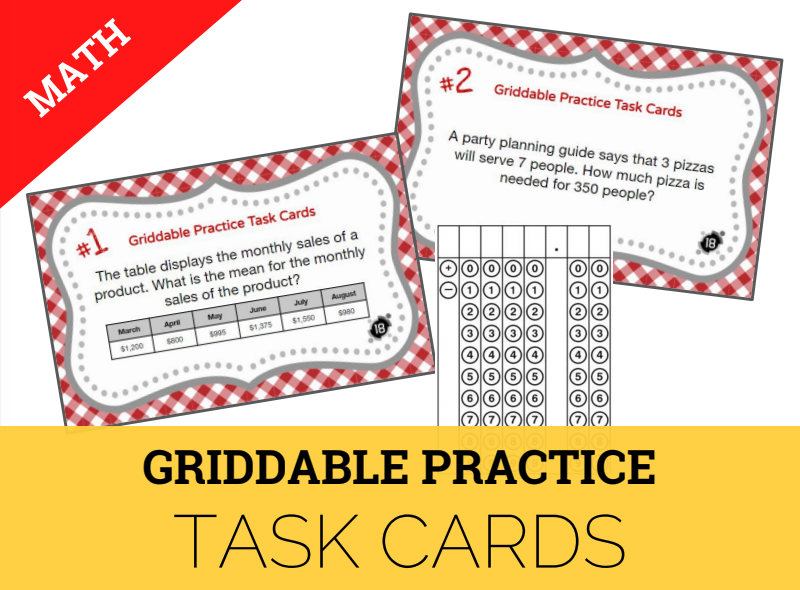Solve problems and record answers in the STAAR griddable format.